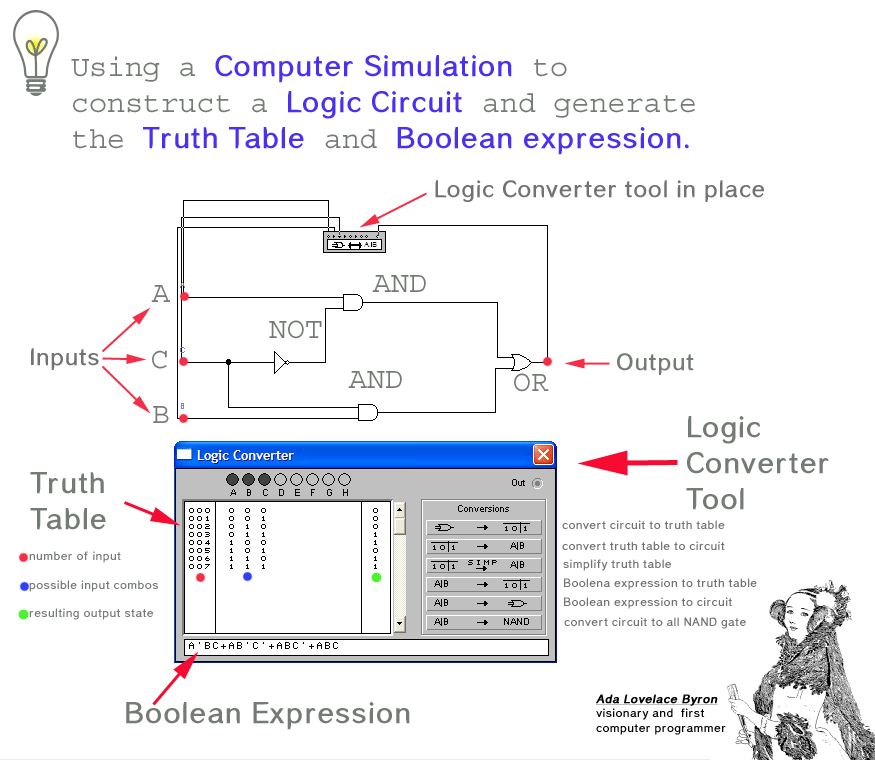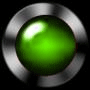I  was paid this Thursday AND my girlfriend's available ....... the date is ON.
The ethanol tank is correct temp.
AND container is in correct position ...... FILL NOW.

That's logic .... one set of conditions allow for some other event to be true.
Logic was developed when Aristotle (384 to 322 BC) wrote a book covering the art of discourse called the Organon (meaning instrument or tool) about the methodology of logic.

In order to control a process (the date) conditions must be met and a final decision made based on the inputs conditions.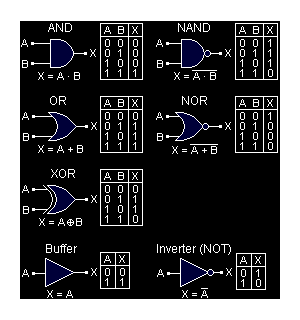In 1854 George Boole (1815-1864) unified algebra and functional logic in his book, The Laws of Thought, by creating Boolean algebra which uses numbers to represent truth values.

Thus "true" is usually represented by "1" and "false" is represented by "0".

Truth Tables  invented by philosopher Ludwig Wittgenstein are a device used to account for all possible logical input states and the logical output states.

In electrical theory a circuit is energized or it is not
1 represents ON    (HI)
0 represents OFF  (LO)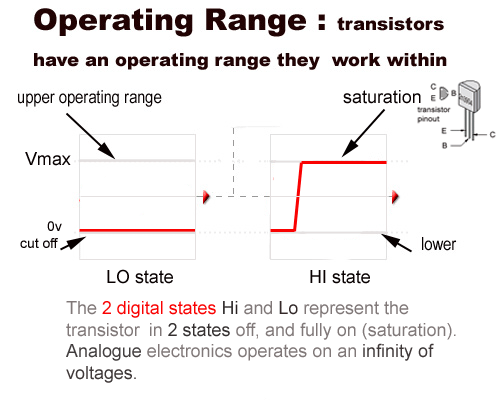Introduction to digital logic gates and the circuits that make them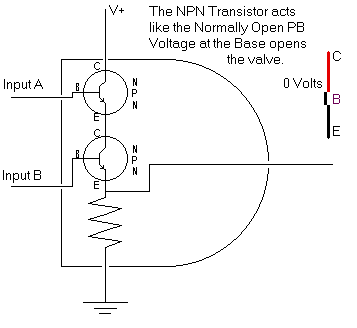This is a digital logic AND gate built from 2 NPN transistors connected in series

When both inputs A AND B go HI (connect to positive) the output goes HI

If one input is Lo then the output is Lo

Both A AND B must be Hi for the output to go Hi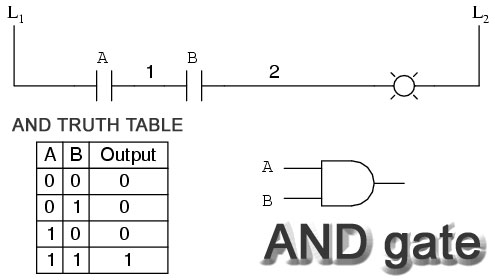The AND gate is like 2 switches in series unless switch A AND B are closed (like the transistors above and below) the load is not energized.
Truth Tables list the possible states that can exist at the input and the resulting logic output.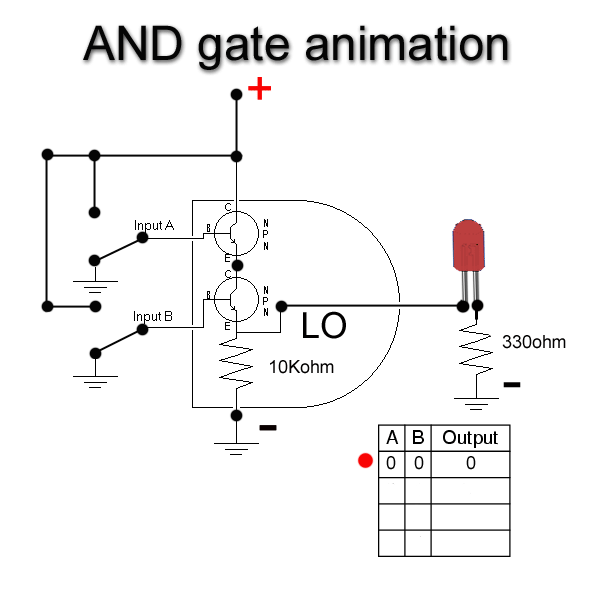Follow this animation several times until you intuitively understand what is happening at the input and the output. The LED will not turn on until the output goes positive Hi to complete its circuit. The only way for the output to go Hi is for both transistors to turn on completing the circuit. For both transistors to turn on both must connect their bases to the Hi input. This allows base current to flow which forward biases the 2    transistors on.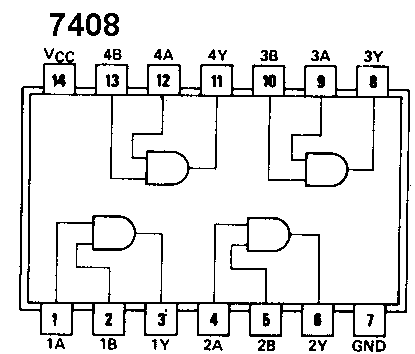The 7408 DIP (dual inline package) 14 pin chip
contains 4 separate AND gates

NOTE: pins 14 and 7 are the source power pins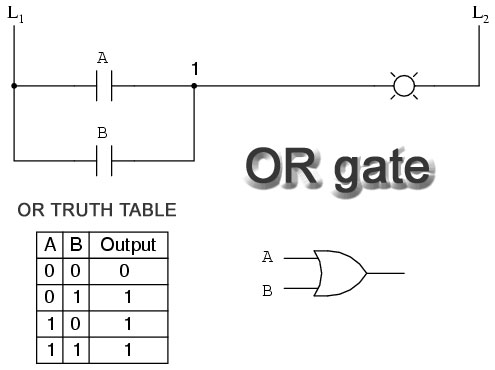Here is an OR gate which sends its output Hi when either input goes Hi

The OR gate is like switches in parallel if either switch is closed the output will go Hi

You might have guessed that the OR gate is constructed using 2 transistors connected in parallel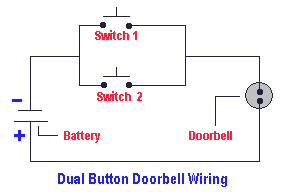Digital OR circuit made from 2 switches in parallel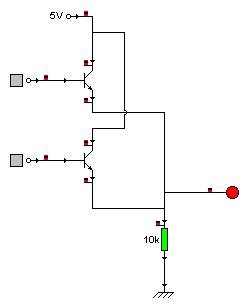Here is the internal construction of an OR gate made of 2 NPN transistors connected in parallel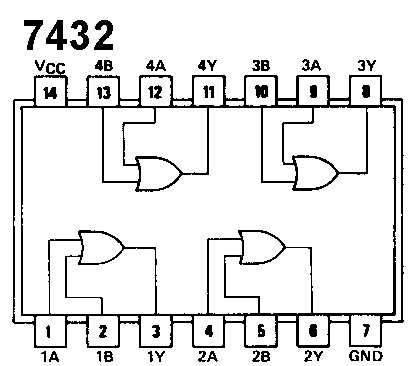The 7432 chip is a 14 pin DIP chip contains 4 OR gates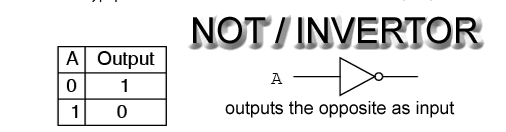Combine the NOT with an AND and you get a NAND

combine the NOT with an OR and you have a NOR

- put 2 invertors together in series is like a double negative in language so the output is the same as the input and the current is amplified
- this NOT NOT correct!!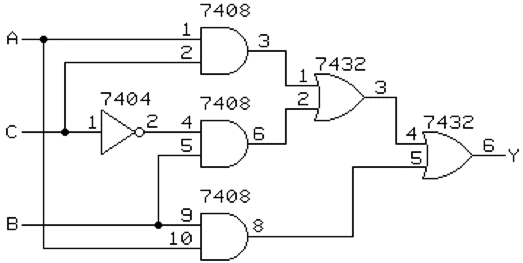This Combinational Logic circuit shows 3 inputs that combine to determine the state of the output which might be a light or motor etc control circuit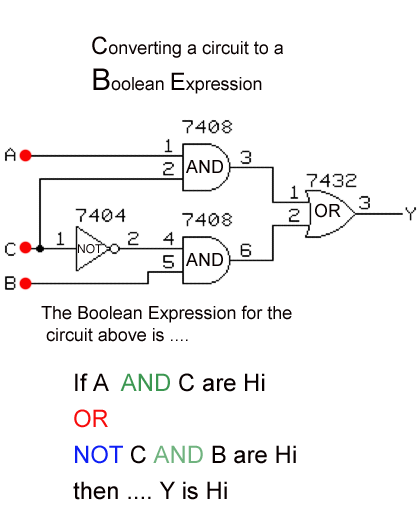below is the same circuit in a simulation with a converter tool attached